# Fast AutoML with FLAML + Ray Tune

FLAML is a lightweight Python library from Microsoft Research that finds accurate machine learning models in an efficient and economical way using cutting edge algorithms designed to be resource-efficient and easily parallelizable. FLAML can also utilize Ray Tune for distributed hyperparameter tuning to scale up these AutoML methods across a cluster.

This blog highlights:

• The need for economical AutoML methods

• Economical AutoML with FLAML

• How to scale FLAML’s optimization algorithms with Ray Tune## LinkThe need of economical AutoML methods

AutoML is known to be a resource and time consuming operation as it involves trials and errors to find a hyperparameter configuration with good performance. Since the space of possible configuration values is often very large, there is a need for an economical AutoML method that can more effectively search them.

The high resource and time consumption of hyperparameter search in AutoML boils down to the following two factors:

1. large number of candidate hyperparameter configurations (trial) needed to find a configuration with good performance

2. high ‘evaluation’ cost of each hyperparameter as the evaluation involves training and validating a machine learning model with the given training data.

To address both of these factors, Microsoft Researchers have developed FLAML (Fast Lightweight AutoML).

WHAT IS FLAML?

FLAML is a newly released library containing state-of-the-art hyperparameter optimization algorithms. FLAML leverages the structure of the search space to optimize for both cost and model performance simultaneously. It contains two new methods developed by Microsoft Research:

• Cost-Frugal Optimization (CFO)

• BlendSearch

Cost-Frugal Optimization (CFO) is a method that conducts its search process in a cost-aware fashion. The search method starts from a low-cost initial point and gradually moves towards a higher cost region while optimizing the given objective (like model loss or accuracy).

Blendsearch is an extension of CFO that combines the frugality of CFO and the exploration ability of Bayesian optimization. Like CFO, BlendSearch requires a low-cost initial point as input if such point exists, and starts the search from there. However, unlike CFO, BlendSearch will not wait for the local search to fully converge before trying new start points.

The economical HPO methods in FLAML are inspired by two key insights:

1. Many machine learning algorithms have hyperparameters that can cause a large variation in the training cost. For example, an XGBoost model with 10 trees will train much quicker than a model with 1000 trees.

2. The “cost” for parameters is often ‘continuous and consistent’ -- evaluating trees=10 is cheaper than evaluating trees=100, which itself is cheaper than evaluating trees=500.

Together, these insights provide useful structural information about the hyperparameters in the cost space. The methods, i.e., CFO and BlendSearch, are able to effectively leverage these insights to reduce the cost incurred along the way without affecting the convergence to the optimal solution.

DOES FLAML WORK?

In the latest AutoML benchmark, FLAML is able to achieve the same or better performance as the state-of-the-art AutoML solutions using only 10% of the computation resource on over 62% of the tasks.

FLAML’s performance is attributed to its economical optimization methods. The new HPO methods (CFO, BlendSearch) leverage the structure of the search space to choose search orders optimized for both good performance and low cost. This can make a big difference in search efficiency under budget constraints.

Figure 1 shows a typical result obtained from FLAML and a state-of-the-art hyperparameter tuning library Optuna for tuning LightGBM with 9-dimensional hyperparameters. You can see that FLAML is able to achieve a better solution in a much shorter amount of time.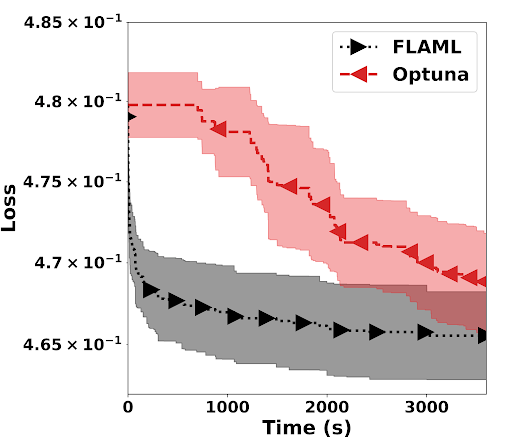Figure 1. Validation loss (1-auc) curve for tuning LightGBM on a classification dataset. The lines and shaded area show the mean and standard deviation of validation loss over 10 runs. Results in this figure are obtained from experiments with 1 cpu (without parallelization).

The following code example shows how to get started with FLAML with just several lines of code (assuming the training dataset is provided and saved as `X_train`, `y_train`). The task is to tune hyperparameters of the LightGBM model with a time budget of 60 seconds.

``````1
2
3
4
5
6
7
from flaml import AutoML
automl = AutoML()
automl.fit(X_train=X_train, y_train=y_train, time_budget=60, estimator_list=['lgbm'])

''' retrieve best model and best configuration found'''
print('Best ML model:', automl.model)
print('Best hyperparameter config:', automl.best_config)``````

In this example, we search over a default search space for LightGBM, which is already provided in FLAML. FLAML provides rich customization options about one's concerned task, such as the learner class, the search space, the evaluation metric, etc.

Now we use a toy example to demonstrate cost-frugal behavior of CFO in tuning XGBoost with two hyperparameters: # of trees and # of leaves.

``````1
2
3
4
5
6
7
8
9
10
11
12
13
14
15
16
17
18
19
20
21
22
23
24
25
'''create an XGBoost learner class with a customized search space'''
from flaml.model import XGBoostSklearnEstimator
from flaml import tune

class MyXGB(XGBoostSklearnEstimator):
​​    '''XGBoostSklearnEstimator with a customized search space'''
@classmethod
def search_space(cls, data_size, **params):
upper = min(2**15, int(data_size))
return {
'n_estimators': {
'domain': tune.lograndint(lower=4, upper=upper),
'low_cost_init_value': 4,
},
'max_leaves': {
'domain': tune.lograndint(lower=4, upper=upper),
'low_cost_init_value': 4,
},
}

'''Use CFO in FLAML to tune XGBoost'''
from flaml import AutoML
automl = AutoML()
automl.fit(X_train=X_train, y_train=y_train, time_budget=15, estimator_list=['my_xgboost'], hpo_method='cfo')``````

### LinkHow CFO and BlendSearch Work

The two GIFs below demonstrate the search trajectory of CFO in the loss and evaluation cost (i.e., the evaluation time ) space respectively. CFO begins with a low-cost initial point (specified through `low_cost_init_value` in the search space) and performs local updates following its randomized local search strategy. With such a strategy, CFO can quickly move toward the low-loss region, showing a good convergence property. Additionally, CFO tends to avoid exploring the high-cost region until necessary. This search strategy is further grounded with a provable convergence rate and bounded cost in expectation.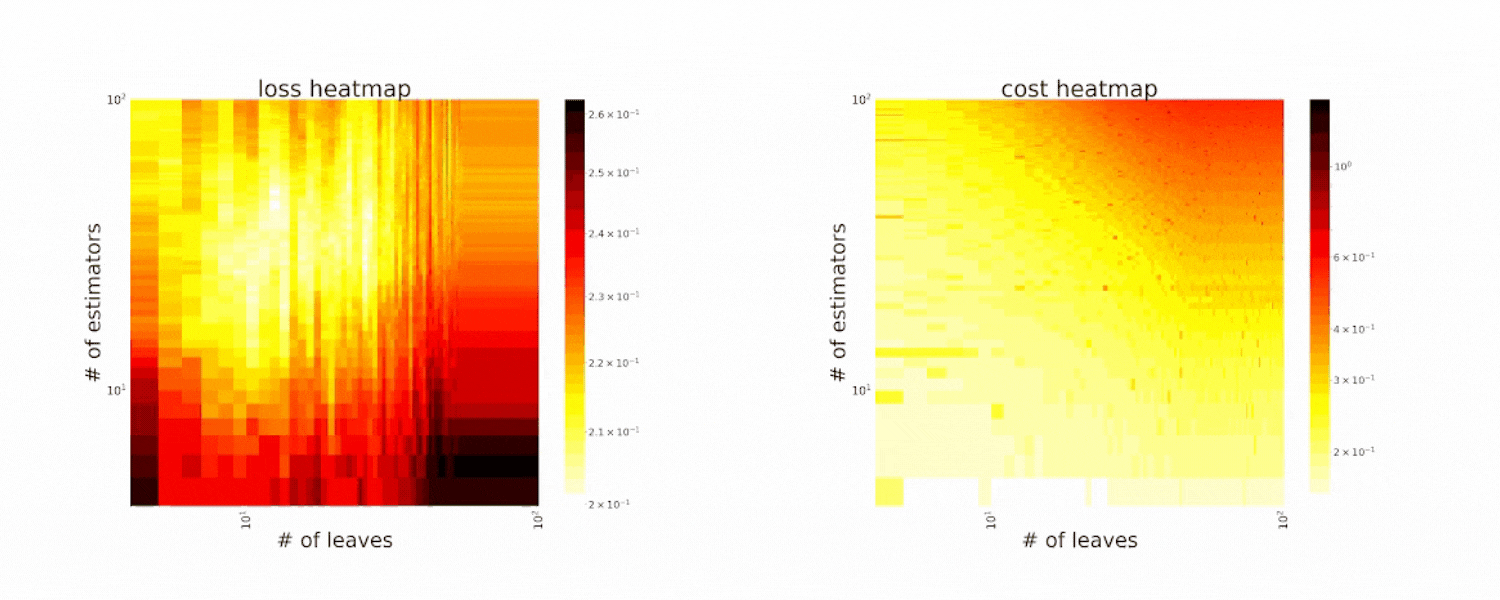Figure 2. CFO in tuning the # of leaves and the # of trees for XGBoost. The two heatmaps show the loss and cost distribution of all configurations. The black dots are the points evaluated in CFO. Black dots connected by lines are points that yield better loss performance when evaluated.

BlendSearch further combines this local search strategy used in CFO with global search. It leverages the frugality of CFO and the space exploration capability of global search methods such as Bayesian optimization. Specifically, BlendSearch maintains one global search model, and gradually creates local search threads over time based on the hyperparameter configurations proposed by the global model. It further prioritizes the global search thread and multiple local search threads depending on their real-time performance and cost. It can further improve the efficiency of CFO in tasks with complicated search space, e.g., a search space that contains multiple disjoint, non-continuous subspaces.

### LinkFLAML vs Bayesian Optimization Performance

Figure 3 shows typical behaviors of the economical HPO methods in FLAML (CFO is labeled `LS’ in this figure) versus a Bayesian Optimization (BO) method for tuning XGBoost with 11 hyperparameters.

From Figure 3(a), we observe that the evaluation time for the proposed configurations in BO can be very long. When the total resource is limited, e.g., 1 cpu-hour (or less), BO is not able to give a satisfying result (Figure 3(b)).

FLAML’s CFO (labeled LS) and BlendSearch have clear advantages in finding good configurations quickly: they are able to concentrate on configurations that have low evaluation time, while navigating ones with good performance, i.e., low loss.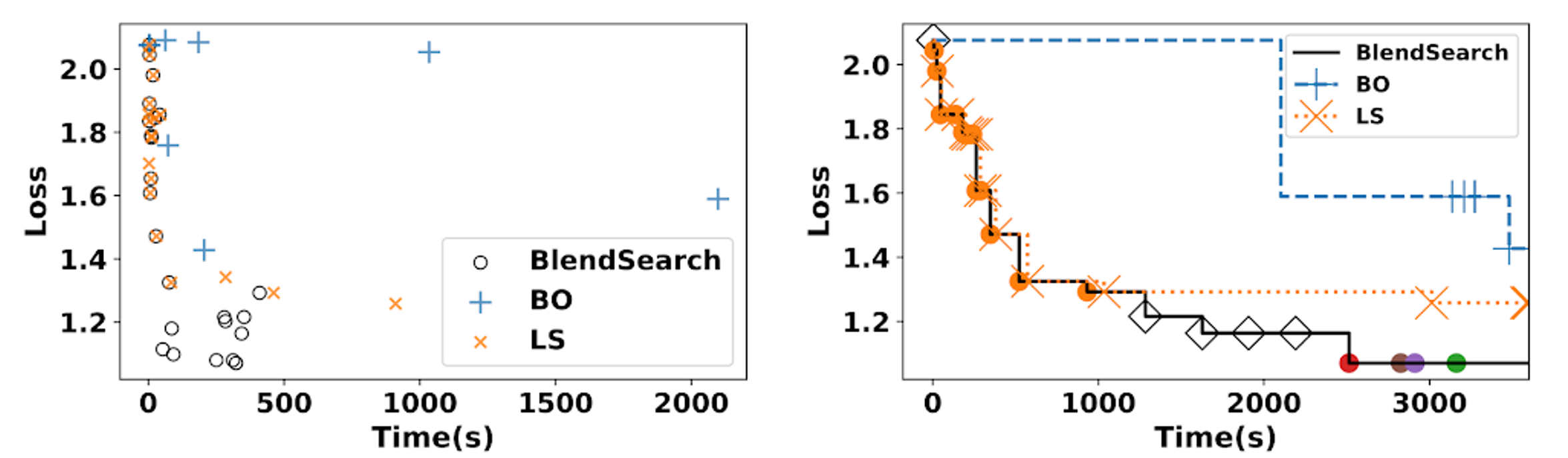Figure 3. (a) is a scatter plot of the hyperparameter configurations proposed by different methods, with x-axis and y-axis being the evaluation time and loss. The evaluation time of a hyperparameter configuration is the time taken for training a machine learning model with the hyperparameter configuration on the training data and validating its performance on a validation dataset. The loss is the validation loss. (b) shows the best loss obtained by different methods over wall-clock time. (image source)

## LinkHow to scale up CFO and BlendSearch with Ray Tune’s distributed tuning

To speed up hyperparameter optimization, you may want to parallelize your hyperparameter search. For example, BlendSearch is able to work well in a parallel setting: It leverages multiple search threads that can be independently executed without obvious degradation of performance. This desirable property is not always true for existing optimization algorithms such as Bayesian Optimization.

To achieve parallelization, FLAML is integrated with Ray Tune. Ray Tune is a Python library that accelerates hyperparameter tuning by allowing you to leverage cutting edge optimization algorithms at scale. Ray Tune also allows you to scale out hyperparameter search from your laptop to a cluster without changing your code. You can either use Ray Tune in FLAML or run the hyperparameter search methods from FLAML in Ray Tune to parallelize your search. The following code example shows the former usage, which is achieved by simply configuring the `n_concurrent_trials` argument in FLAML.

``````1
2
3
4
5
'''Use BlendSearch for hyperparameter search, and Ray Tune for parallelizing concurrent trials (when n_concurrent_trials > 1) in FLAML to tune XGBoost'''
from flaml import AutoML
automl = AutoML()
automl.fit(X_train=X_train, y_train=y_train, time_budget=15, estimator_list=['my_xgboost'], hpo_method='bs', n_concurrent_trials=8)``````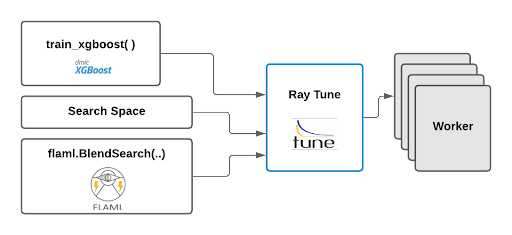The code below shows the latter usage, an end-to-end example of how to use BlendSearch with Ray Tune.

``````1
2
3
4
5
6
7
8
9
10
11
12
13
14
15
16
17
18
19
20
21
22
23
24
25
26
27
28
29
30
31
32
33
34
35
36
37
38
39
40
41
42
43
44
45
46
from ray import tune
from flaml import CFO, BlendSearch
import time

def training_func(config):
'''evaluate a hyperparameter configuration'''
# we use a toy example with 2 hyperparameters
metric = (round(config['x'])-85000)**2 - config['x']/config['y']

# usually the evaluation takes a non-neglible cost
# and the cost could be related to certain hyperparameters
# in this example, we assume it's proportional to x
time.sleep(config['x']/100000)
# use tune.report to report the metric to optimize
tune.report(metric=metric)

# provide the search space
search_space = {
'x': tune.lograndint(lower=1, upper=100000),
'y': tune.randint(lower=1, upper=100000)
}

# provide the low cost partial config
low_cost_partial_config={'x':1}

# set up BlendSearch
blendsearch = BlendSearch(
metric="metric", mode="min",
space=search_space,
low_cost_partial_config=low_cost_partial_config)

blendsearch.set_search_properties(config={"time_budget_s": 60})

analysis = tune.run(
training_func,    # the function to evaluate a config
config=search_space,
metric='metric',    # the name of the metric used for optimization
mode='min',         # the optimization mode, 'min' or 'max'
num_samples=-1,    # the maximal number of configs to try, -1 means infinite
time_budget_s=60,   # the time budget in seconds
local_dir='logs/',  # the local directory to store logs
search_alg=blendsearch  # or cfo
)

print(analysis.best_trial.last_result)  # the best trial's result
print(analysis.best_config)  # the best config``````

Other key Ray Tune features include:

• Automatic integration with experiment tracking tools like Tensorboard and Weights/Biases

• Support for GPUs

• Early stopping

• A scikit-learn API to easily integrate with XGBoost, LightGBM, Scikit-Learn, etc.

We have conducted an experiment to check how well BlendSearch stacks up to Optuna (with multivariate TPE sampler) and random search in a highly parallelized setting. We have used a subset of 12 datasets from the AutoML Benchmark. Each optimization run was conducted with 16 trials in parallel for 20 minutes, using 3-fold cross-validation, using ROC-AUC (weighted one-vs-rest for multiclass datasets). The runs were repeated three times with different random seeds. Reproduction code can be found here.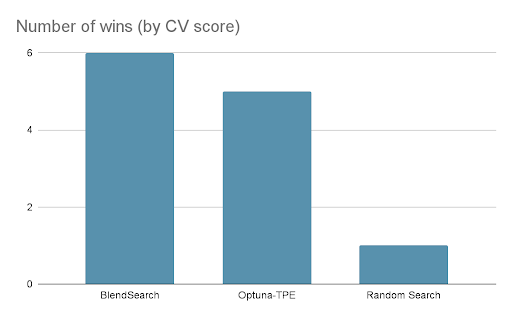BlendSearch achieved the best cross-validation score in 6 out of 12 datasets. Furthermore, BlendSearch had an average of 2.52% improvement over random search, compared to Optuna’s 1.96%. It is worth noting that BlendSearch was using univariate Optuna-TPE as its global searcher - using multivariate TPE would most likely improve the scores further.In addition, thanks to its cost-frugal approach, BlendSearch evaluated, on average, twice the number of trials than the other searchers in the same time limit. This shows that the gap between BlendSearch and the other algorithms will increase with bigger time budgets.